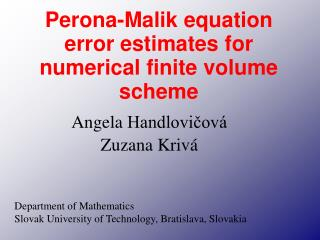Download PresentationPerona-Malik equation error estimates for numerical finite volume scheme

# Perona-Malik equation error estimates for numerical finite volume scheme - PowerPoint PPT PresentationDownload Presentation## Perona-Malik equation error estimates for numerical finite volume scheme

- - - - - - - - - - - - - - - - - - - - - - - - - - - E N D - - - - - - - - - - - - - - - - - - - - - - - - - - -
##### Presentation Transcript

1. AngelaHandlovičová Zuzana Krivá Perona-Malik equation error estimates for numerical finite volume scheme Department of Mathematics Slovak University of Technology, Bratislava, Slovakia

2. Initial noisy image Smooth initial noisy image and preserve edges

3. Perona-Malik Equation

4. g(s) is Lipschitz continuous decreasing function, g(0)=1, 0< g(s) 0 for s is a smoothing kernel with compact support for with Dirac function at point x u0(x) is initial condition Perona-Malikequation - properties of data and

5. Results obtained for finite volume numerical scheme • Existence of weak solutions and regularity-Catté, Lions, Morel, Coll (1992) • Convergence of semi-implicit finite volume numerical scheme – Mikula Ramarossy (2001) • Convergence of explicit finite volume scheme Krivá (2003) • Error estimates for semi-implicit FV scheme Handlovičová, Krivá (2005) • Error estimates for explicit scheme H,K (2005)

6. Finite volume p…volume of measure m(p), with representative point xp N(p) …neighbors of p epq…common edge between volumes p and q of measure m(epq) dpq:=|xp-xq|, Tpq :=m(epq)/d pq upn …numerical solution

7. Explicit scheme

8. Semi - implicit scheme

9. Regularity of the solution

10. For explicit scheme Error estimates For semi -implicit scheme

11. AngelaHandlovičová Zuzana Krivá Department of Mathematics Slovak University of Technology, Bratislava, Slovakia Thank you